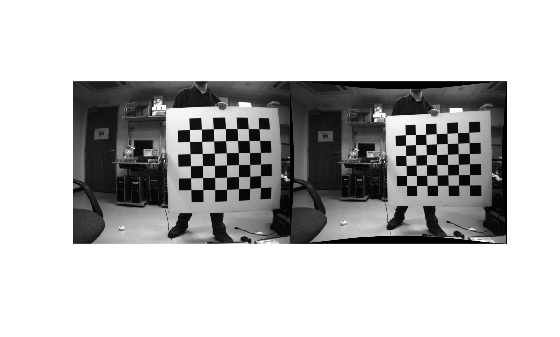Import Camera Intrinsic Parameters from ROS

The ROS camera calibration package estimates camera intrinsic parameters using the OpenCV camera calibration tools . After calibrating a camera in ROS, you can import its intrinsic parameters to a YAML file using the camera calibration parser in ROS. To use the calibrated camera with Computer Vision Toolbox™ functions, such as undistortImage, you must read the camera parameters from the YAML file and then convert them into a cameraIntrinsics object using cameraIntrinsicsFromOpenCV.

Note: The cameraIntrinsicsFromOpenCV function supports importing camera intrinsic parameters for only those pinhole camera models that use the ROS plumb-bob distortion model.

Read Camera Intrinsic Parameters from a ROS YAML File

Read the camera parameters stored in cameraParams.yaml using the helper function helperReadYAML.

Create cameraIntrinsics Object Using cameraIntrinsicsFromOpenCV

Use the cameraIntrinsicsFromOpenCV function to create a cameraIntrinsics object from the camera matrix and the distortion coefficients.

imageSize = [intrinsicsParams.image_height intrinsicsParams.image_width];
intrinsicMatrix = intrinsicsParams.camera_matrix;
distortionCoefficients = intrinsicsParams.distortion_coefficients;

intrinsicsObj = cameraIntrinsicsFromOpenCV(intrinsicMatrix,distortionCoefficients,imageSize);

Undistort Image

Use the imported camera intrinsics with undistortImage to undistort an image captured using the calibrated camera.

imageName = fullfile(toolboxdir('vision'),'visiondata','calibration','stereo','left','left01.png');

% Undistort the image.
J = undistortImage(I,intrinsicsObj,'OutputView','full');

% Display the result.
figure
montage({I,J})Supporting Functions

The helperReadYAML function reads the monocular camera parameters from the input YAML file that was exported from ROS.

% helperReadYAML reads a ROS YAML file, filename, and returns a structure
% with these fields: image_width, image_height, camera_name,
% camera_matrix, distortion_model, distortion_coefficients,
% rectification_matrix, and projection_matrix. These fields are stored
% in the YAML file colon separated from their values in different lines.

f = fopen(filename,'r');
stringFields = {'camera_name','distortion_model'};

while ~feof(f)

if isEmptyLine
continue
end

if ~isempty(value)
% Convert all values to numbers except for known string
% fields.
if ~any(contains(name, stringFields))
value = str2num(value); %#ok
end
else
% An empty value in ROS YAML files indicates a matrix in
% upcoming lines. Read the matrix from the upcoming lines.
end

% Store post-processed value.
cameraParams.(name) = value;
end

fclose(f);
end

The helperReadYAMLMatrix function reads the rows, columns and data fields of a matrix in the ROS YAML file.

%   helperReadYAMLMatrix reads a matrix from the ROS YAML file. A matrix in
%   a ROS YAML file has three fields: rows, columns and data. rows and col
%   describe the matrix size. data is a continguous array of the matrix
%   elements in row-major order. This helper function assumes the presence
%   of all three fields of a matrix to return the correct matrix.

numRows = 0;
numCols = 0;
data = [];

% Read numRows, numCols and matrix data.
while ~feof(f)

if isEmptyLine
continue
end

switch name
case 'rows'
numRows = str2num(value); %#ok
case 'cols'
numCols = str2num(value); %#ok
case 'data'
data    = str2num(value); %#ok

% Terminate the while loop as data is the last
% field of a matrix in the ROS YAML file.
break
otherwise
% Terminate the while loop if any other field is
% encountered.
break
end
end

if numel(data) == numRows*numCols
% Reshape the matrix using row-major order.
matrix = reshape(data,[numCols numRows])';
end
end

line = fgetl(f);

% Trim leading and trailing whitespaces.
line = strtrim(line);

if isempty(line) || line(1)=='#'
% Empty line or comment.
name = '';
value = '';
isEmptyLine = true;
else
% Split the line to get name and value.
c = strsplit(line,':');
assert(length(c)==2,'Unexpected file format')

name = c{1};
value = strtrim(c{2}); % Trim leading whitespace.
isEmptyLine = false;
end
end